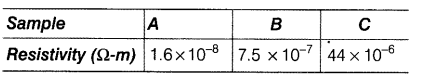# Following table gives the resistivity of three samples in ohm-m

Following table gives the resistivity of three samples in ohm-m.Which of them is a good conductor and which of them is an insulator, why?

A material having low resistivity is a good conductor. Since, resistivity of sample A is the least among all other materials, so sample A is a good conductor. A material having high value of resistivity is an insulator. Therefore, sample C is having high value of resistivity among all materials. So, sample C is an insulator.